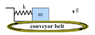# SHO on a conveyor belt

cbjewelz
This one is killing me.

## Homework Statement

A SHO is resting on a horizontal surface with which it has static and
kinetic friction coefficients us and uk . We have k for the spring constant
and m for the mass. The surface is actually part of a conveyor belt
that is maintained at a speed u whatever the behavior of the SHO. (speed is to the right)
see figure
Assume that the mass of the SHO is initially at rest at its equilibrium
position. The motion can be divided into distinct regimes, depending
upon whether there is slipping or not. You are to begin with the first
regime.

(a) Derive expressions for the displacement and velocity as a function
of time.
(b) Derive the condition under which the SHO will oscillate sinu-
soidally forever.
Consider the situation under which the mass can experience static
friction.
(c) At what time t1 does the SHO lose its sinusoidal behavior and
sticks? Express t1 in terms of the given parameters.
(d) What is the displacement at the point when the mass sticks? Ex-
press your answer in terms of u, ?k , g, and the angular frequency
?0 of the SHO.
(e) What is the motion of mass when it sticks?
(f ) What is the displacement when the mass begins to slip?

2. The attempt at a solution
I'm really only having trouble with the first part. I'm thinking that the conveyor belt picks up the mass due to static friction and carries it with constant speed until kx overcomes static friction at which point there is slipping, etc. Is this correct? and if so how do we explain how it is initially put into motion because we cannot have an instantaneous force brining the mass to u.

Thanks

#### Attachments

•Picture 1.png
2.7 KB · Views: 429
Last edited:

cbjewelz
I don't know why this was moved to the introductory physics forum. This problem involves multiple differential equations that must be solved with considerations of slipping and frictional forces included.

denverdoc
From the reading of the problem, I don't think it says what you suggest for parts 1) & 2) instead, that the mass is slipping constantly.

tim_lou
I don't think problems like these can be solved using the usual differential equation method.

rather, you should try to explain the motion and connect the pieces together.

first imagine the mass is stationary, it will move at constant speed with the conveyor belt as long as the force exerted by the spring doesn't exceed the static friction. once the spring is pulled hard enough, you'll get some motion. sooner or later, the mass will come around, at some instant, it will move at the same velocity as the conveyor belt, and static friction kicks in, depending on the force at that instance, you will have a couple different situations. you just have to solve for the motions individually and paste them together.

Last edited: